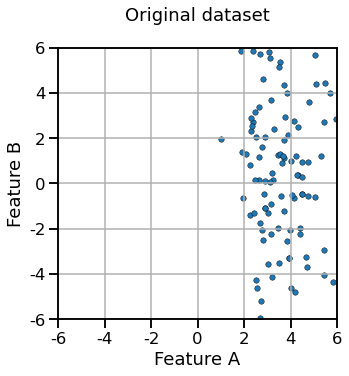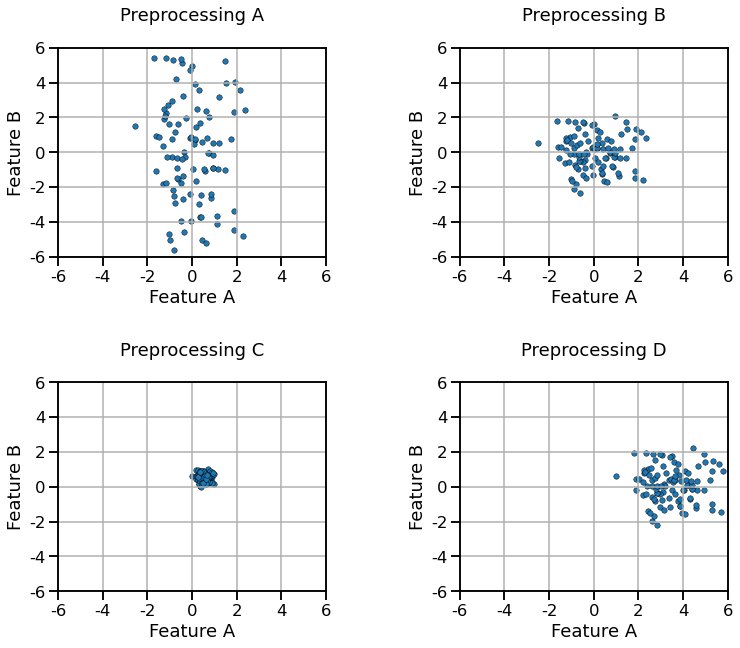# ✅ Quiz M1.02#

Question

Why do we need two sets: a train set and a test set?

• a) to train the model faster

• b) to validate the model on unseen data

• c) to improve the accuracy of the model

Question

The generalization performance of a scikit-learn model can be evaluated by:

• a) calling fit to train the model on the training set, predict on the test set to get the predictions, and compute the score by passing the predictions and the true target values to some metric function

• b) calling fit to train the model on the training set and score to compute the score on the test set

• c) calling cross_validate by passing the model, the data and the target

• d) calling fit_transform on the data and then score to compute the score on the test set

Question

When calling cross_validate(estimator, X, y, cv=5), the following happens:

• a) X and y are internally split five times with non-overlapping test sets

• b) estimator.fit is called 5 times on the full X and y

• c) estimator.fit is called 5 times, each time on a different training set

• d) a Python dictionary is returned containing a key/value containing a NumPy array with 5 scores computed on the train sets

• e) a Python dictionary is returned containing a key/value containing a NumPy array with 5 scores computed on the test sets

We define a 2-dimensional dataset represented graphically as follows:Question

If we process the dataset using a StandardScaler with the default parameters, which of the following results do you expect:• a) Preprocessing A

• b) Preprocessing B

• c) Preprocessing C

• d) Preprocessing D

Question

Look at the plots and the answers of the previous question. A StandardScaler transformer with the default parameter:

• a) transforms the features so that they have similar ranges

• b) transforms the features to lie in the [0.0, 1.0] range

• c) transforms feature values that were originally positive-only into values that can be negative or positive

• d) can help logistic regression converge faster (fewer iterations)

Question

Cross-validation allows us to:

• a) train the model faster

• b) measure the generalization performance of the model

• c) estimate the variability of the generalization score

Question

make_pipeline (as well as Pipeline):

• a) runs a cross-validation using the transformers and predictor given as parameters

• b) combines one or several transformers and a predictor

• c) tries several models at the same time

• d) plots feature histogram automatically